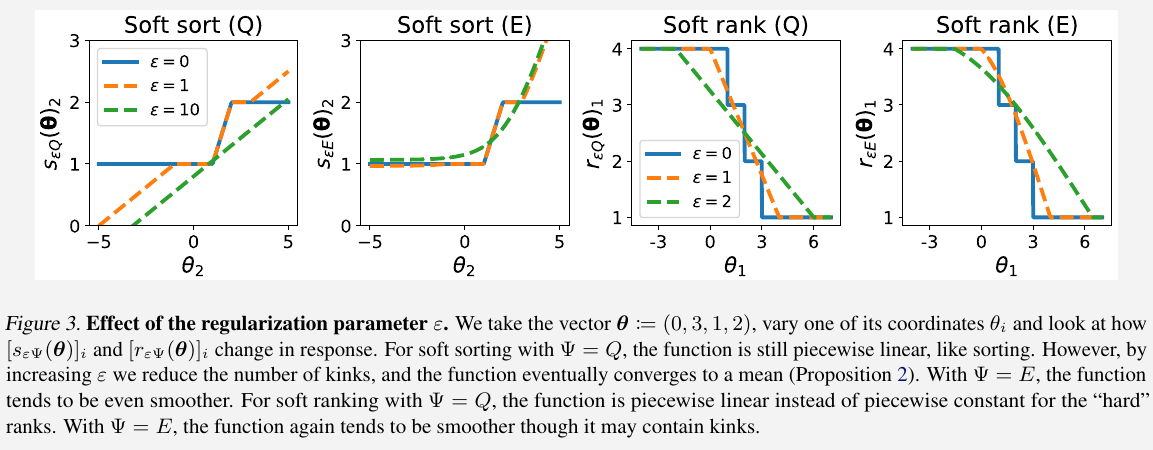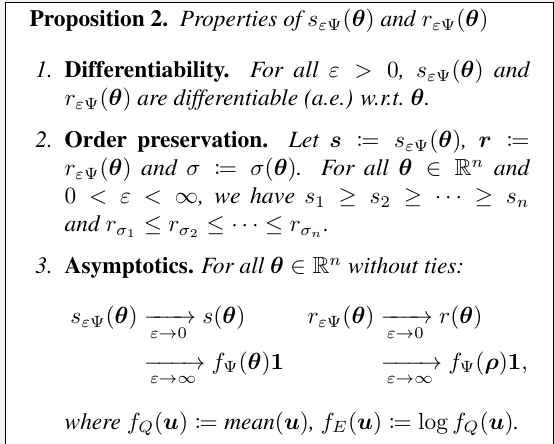# Differentiable Sorting and Ranking

##### Posted on Nov 04, 2022 (Update: Nov 14, 2022)
Tags: Sort, Rank, Linear Programming

## Sorting and ranking as linear programs

Denote the set of $n!$ permutations of $[n]$ by $\Sigma$.

For all $\theta\in \IR^n$ and $\rho:=(n, n-1,\ldots, 1)$, we have

\begin{align*} \sigma(\theta) &= \argmax_{\sigma\in\Sigma} \langle \theta_\sigma, \rho\rangle\\ r(\theta) &= \argmax_{\pi\in\Sigma}\langle \theta, \rho_\pi\rangle \end{align*}

To obtain continuous optimization problems, introduce the permutahedron induced by a vector $w\in\IR^n$, the convex hull of permutations of $w$:

$\cP(w) := \conv(\{w_\sigma:\sigma\in\Sigma\})\subset \IR^n$

A well-known object in combinatorics: the permutahedron of $w$ is a convex polytope, whose vertices correspond to permutations of $w$.

Then one can derive linear programming formulations of sorts and ranks.

For all $\theta\in \IR^n$ and $\rho:=(n, n-1,\ldots, 1)$, we have

\begin{align*} s(\theta) &=\argmax_{y\in\cP(\theta)} \langle y, \rho\rangle\\ r(\theta) &=\argmax_{y\in\cP(\theta)} \langle y, -\theta\rangle \end{align*}

## Differentiable sorting and ranking

Add $Q(\mu) = \frac 12\Vert \mu\Vert_2^2$ to the linear program over the permutahedron,

$P_Q(z, w):=\argmax_{\mu\in\cP(w)} \langle z,\mu\rangle -Q(\mu) = \argmin_{\mu\in \cP(w)}\frac 12\Vert \mu-z\Vert^2$

Also consider entropic regularization $E(\mu)=\langle \mu, \log\mu-1\rangle$,

$P_E(z,w):=\log\argmax_{\mu\in \cP(e^w)} \langle z, \mu\rangle -E(\mu) = \log\argmin_{\mu\in \cP(e^w)} KL(\mu, e^z)$

Choose $(z, w)=(\rho, \theta)$, define the $\Psi$-regularized soft sort

$s_{\varepsilon \Psi}(\theta) = P_{\varepsilon \Psi}(\rho, \theta) = P_{\Psi}(\rho/\varepsilon, \theta)$Published in categories Note